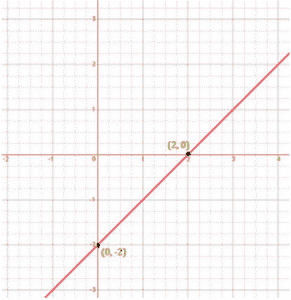Newbie

# Draw the graph of each of the following linear equations in two variables:(ii) x–y = 2. Q.1(2)

• 0

Sir please help me to solve the ncert class 9th solution of chapter linear equations in two variables .How I solve this question of exercise 4.3 question number 1(2). Find the simplest and easiest solution of this question.Draw the graph of each of the following linear equations in two variables:(ii) x–y = 2.

Share

1. Solution:

To draw a graph of linear equations in two variables, let us find out the points to plot.

To find out the points, we have to find the values which x and y can have, satisfying the equation.

Here,

x–y = 2

Substituting the values for x,

When x = 0,

x–y = 2

0 – y = 2

y = – 2

When x = 2,

x–y = 2

2–y = 2

– y = 2–2

–y = 0

y = 0

 x y 0 – 2 2 0

The points to be plotted are (0, – 2) and (2, 0)• 0